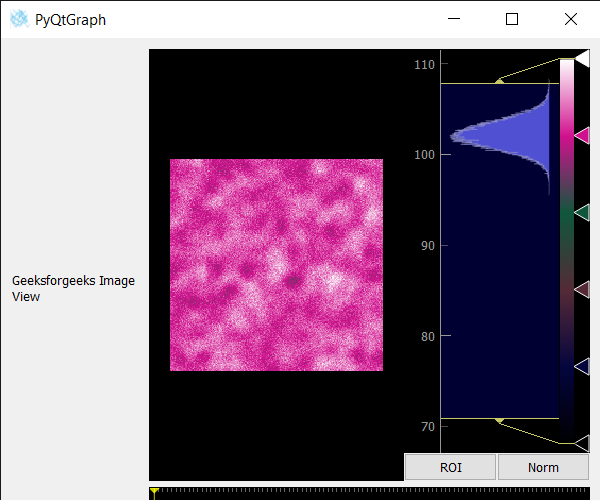Related Articles

# PyQtGraph – Setting Image to Image View

• Last Updated : 25 Oct, 2020

In this article, we will see how we can set an image to the image view object in PyQTGaph. PyQtGraph is a graphics and user interface library for Python that provides functionality commonly required in designing and science applications. Its primary goals are to provide fast, interactive graphics for displaying data (plots, video, etc.). Widget used for display and analysis of image data. Implements many features like displaying 2D and 3D image data. For 3D data, a z-axis slider is displayed allowing the user to select which frame is displayed. Displays histogram of image data with movable region defining the dark/light levels, editable gradient provides a color lookup table, frame slider may also be moved using left/right arrow keys as well as pgup, pgdn, home, and end.

We can create an image view with the help of the command given below

```# creating a pyqtgraph image view object
imv = pg.ImageView()
```

In order to do this we use setImage method with the image view object
Syntax : imv.setImage(img)
Argument : It takes numpy ndarray as argument
Return : It returns None

Below is the implementation.

## Python3

 `# importing Qt widgets``from` `PyQt5.QtWidgets ``import` `*`` ` `# importing system``import` `sys`` ` `# importing numpy as np``import` `numpy as np`` ` `# importing pyqtgraph as pg``import` `pyqtgraph as pg``from` `PyQt5.QtGui ``import` `*``from` `PyQt5.QtCore ``import` `*`` ` ` ` `# Image View class``class` `ImageView(pg.ImageView):`` ` `    ``# constructor which inherit original``    ``# ImageView``    ``def` `__init__(``self``, ``*``args, ``*``*``kwargs):``        ``pg.ImageView.__init__(``self``, ``*``args, ``*``*``kwargs)`` ` ` ` `class` `Window(QMainWindow):`` ` `    ``def` `__init__(``self``):``        ``super``().__init__()`` ` `        ``# setting title``        ``self``.setWindowTitle(``"PyQtGraph"``)`` ` `        ``# setting geometry``        ``self``.setGeometry(``100``, ``100``, ``600``, ``500``)`` ` `        ``# icon``        ``icon ``=` `QIcon(``"skin.png"``)`` ` `        ``# setting icon to the window``        ``self``.setWindowIcon(icon)`` ` `        ``# calling method``        ``self``.UiComponents()`` ` `        ``# showing all the widgets``        ``self``.show()`` ` `        ``# setting fixed size of window``        ``self``.setFixedSize(QSize(``600``, ``500``))`` ` `    ``# method for components``    ``def` `UiComponents(``self``):`` ` `        ``# creating a widget object``        ``widget ``=` `QWidget()`` ` `        ``# creating a label``        ``label ``=` `QLabel(``"Geeksforgeeks Image View"``)`` ` `        ``# setting minimum width``        ``label.setMinimumWidth(``130``)`` ` `        ``# making label do word wrap``        ``label.setWordWrap(``True``)`` ` `        ``# setting configuration options``        ``pg.setConfigOptions(antialias``=``True``)`` ` `        ``# creating image view view object``        ``imv ``=` `ImageView()`` ` `        ``# Create random 3D data set with noisy signals``        ``img ``=` `pg.gaussianFilter(np.random.normal(``            ``size``=``(``200``, ``200``)), (``5``, ``5``)) ``*` `20` `+` `100`` ` `        ``# setting new axis to image``        ``img ``=` `img[np.newaxis, :, :]`` ` `        ``# decay data``        ``decay ``=` `np.exp(``-``np.linspace(``0``, ``0.3``, ``100``))[:, np.newaxis, np.newaxis]`` ` `        ``# random data``        ``data ``=` `np.random.normal(size``=``(``100``, ``200``, ``200``))``        ``data ``+``=` `img ``*` `decay``        ``data ``+``=` `2`` ` `        ``# adding time-varying signal``        ``sig ``=` `np.zeros(data.shape[``0``])``        ``sig[``30``:] ``+``=` `np.exp(``-``np.linspace(``1``, ``10``, ``70``))``        ``sig[``40``:] ``+``=` `np.exp(``-``np.linspace(``1``, ``10``, ``60``))``        ``sig[``70``:] ``+``=` `np.exp(``-``np.linspace(``1``, ``10``, ``30``))`` ` `        ``sig ``=` `sig[:, np.newaxis, np.newaxis] ``*` `3``        ``data[:, ``50``:``60``, ``30``:``40``] ``+``=` `sig`` ` `        ``# setting image to image view``        ``# Displaying the data and assign each frame a time value from 1.0 to 3.0``        ``imv.setImage(data, xvals``=``np.linspace(``1.``, ``3.``, data.shape[``0``]))`` ` `        ``# Set a custom color map``        ``colors ``=` `[``            ``(``0``, ``0``, ``0``),``            ``(``4``, ``5``, ``61``),``            ``(``84``, ``42``, ``55``),``            ``(``15``, ``87``, ``60``),``            ``(``208``, ``17``, ``141``),``            ``(``255``, ``255``, ``255``)``        ``]`` ` `        ``# color map``        ``cmap ``=` `pg.ColorMap(pos``=``np.linspace(``0.0``, ``1.0``, ``6``), color``=``colors)`` ` `        ``# setting color map to the image view``        ``imv.setColorMap(cmap)`` ` `        ``# Creating a grid layout``        ``layout ``=` `QGridLayout()`` ` `        ``# minimum width value of the label``        ``label.setFixedWidth(``130``)`` ` `        ``# setting this layout to the widget``        ``widget.setLayout(layout)`` ` `        ``# adding label in the layout``        ``layout.addWidget(label, ``1``, ``0``)`` ` `        ``# plot window goes on right side, spanning 3 rows``        ``layout.addWidget(imv, ``0``, ``1``, ``3``, ``1``)`` ` `        ``# setting this widget as central widget of the main widow``        ``self``.setCentralWidget(widget)`` ` ` ` `# create pyqt5 app``App ``=` `QApplication(sys.argv)`` ` `# create the instance of our Window``window ``=` `Window()`` ` `# start the app``sys.exit(App.``exec``())`

Output :Attention geek! Strengthen your foundations with the Python Programming Foundation Course and learn the basics.

To begin with, your interview preparations Enhance your Data Structures concepts with the Python DS Course. And to begin with your Machine Learning Journey, join the Machine Learning – Basic Level Course

My Personal Notes arrow_drop_up